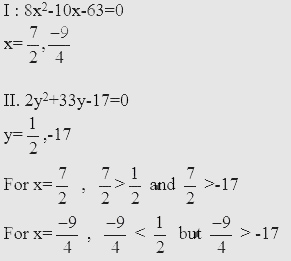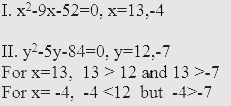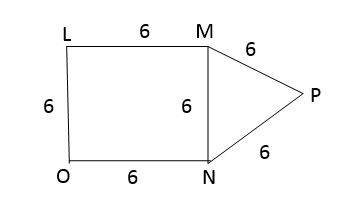# Sample Aptitude Questions of Flextronics

DIRECTIONS for the questions 1 to 2: Solve the following question and mark the best possible option
1. I. 8x2 – 10x – 63 = 0
II. 2y2 + 33y - 17 = 0
1. x < = y
2. x >= y
3. x < y
4. x > y
5. Relationship between x and y cannot be established.Hence no relationship can be established between x & y.
1. x2 - 9x - 52 = 0
II. y2 - 5y - 84 = 0
1. x < y
2. x > y
3. x <= y
4. Relationship between x and y cannot be established.
5. x >= yHence no relationship can be established between x & Y
DIRECTIONS for the questions 3 to 4: In the following series, one of the terms given is wrong. Find that term and mark that as your answer.
2. 2 3 10 39 178 885
1. 855
2. 178
3. 10
4. 304
5. 39
The logic is ×1+1, ×2+4, ×3+9, ×4+16, ×5+25,….
So following the logic we get 178 is wrong instead it should be 172. …..
3. 139 150 149 174 223 304
1. 149
2. 150
3. 174
4. 304
5. 223
The difference should be, +12,+32,+52,+72,+92,….
So the correct series is 139,140,149,174,223,304.
4. The figure given below, MNP is an equilateral triangle and LMNO is a square with side 6 cm. What is the area of the pentagon PMLON1. 72√3 cm2
2. 36√3 cm2
3. 6( 3 + √3 ) cm2
4. 9( 4 + √3 ) cm2
5. 48√3 cm2
Area of square LMNO = 36 cm2
MNP is an equilateral traingle with side MN = 6 cm
Area of MNP = √3/4 * 62 = 9√3 cm2
Area of Pentagon LMPNO = Area of Triangle MNP + Area of square LMNO = ( 36 + 9√3 ) = 9( 4 + 9√3) cm2
5. What is the total cost of construction of a 1m wide path outside the rectangular plot along all the four sides, given the following 2 condtitions :
I. Perimeter of the plot in 260 m and cost of construction of the path is Rs. 850 per m2
II. Area of the plot is 4125 m2 and cost of construction of the path is Rs. 850 per 2
1. The data in statement II alone are sufficient the question the question, while the data in statement I alone are not sufficient to answer the question.
2. The data in both the statements I & II are insufficient to answer the question.
3. The data either in statement I alone or in II statement alone are sufficient to answer the question.
4. The data in both the statements I & II together are necessary to answer the question.
5. The data in Statement I alone are sufficient to the question, while the data in statement II alone are not sufficient to answer the question.
By using both the statements together ,
The perimeter of plot ,2( L + B ) = 260m Area of plot, L × B = 4125 m2,
Solving these two equations :
we can find that the length of the plot is 75m and the breadth of the plot is 55m.
Now we can easily find the area of the path all around the rectangular plot.
Also the cost of construction is same as Rs 850/m2. Hence we can find the total cost of construction of the path.
6. In the stream running at 3 kmph, a motorboat goes 12 km upstream and returns back to the starting point in 128 minutes. What is the speed of the boat in still water? (in kmph)
1. 20
2. 12
3. 15
4. 25
5. 10
Let speed of boat in still water = x km/hr
Speed of stream = 3 km/hr
Speed downstream = ( x + 3 ) km/hr,
Speed upstream = ( x - 3 ) km/hr
Therefore according to question,
12/( x - 3 ) + 12/( x + 3 ) = 128/60
Solving ,we get x = 12 km/hr.
7. The respective ratio between ages of P and Q is 11: 7. The respective ratio between P’s age 2 years ago and Q’s age 2 years hence is 7:5. Find the respective ratio between P’s age 7 years hence and Q’s age 7 years ago?
1. 17:9
2. 15:7
3. 19:13
4. 13:5
5. 17:7
Let the present ages of P and Q be 11x,7x respectively. According to the question => (11x - 2)/(7x + 2) = 7/5 => x = 4
Ratio of P’s age 7 years hence & Q’s age 7 years ago = (11 × 4 + 7)/(7 × 4 - 7) = 51/21 = 17/7
8. A jar was containing 60 liters of mixture of milk and water in the ratio 7:5. From this jar 12 litres of mixtures was taken out and 8 litres of pure milk was added. What is the respective ratio of milk and water in the mixture after the final operation?
1. 9:5
2. 4:3
3. 9:7
4. 6:5
5. 9:3
Let n be the mixture quantity of milk = 7/12 × 60 Ltr. = 35 Ltr.
Quantity of water = 5/12 × 60 Ltr. = 25 Ltr.
12 Ltr. of mixture removed contains milk = 7/12 × 12 Ltr. = 7 Ltr and water = 5/12 × 12 Ltr = 5 Ltr
After adding 8Ltr of pure milk,
Net milk in the mixture = 35 - 7 + 8 = 36 Ltr.
Net water in the mixture = 25 - 5 = 20 Ltr.
So the required ratio of milk and water now = 36/20 = 9:5
9. A project manager estimated that he would complete the project in time if he hires 42 people for 38 days. At the end of 30 days he realized that only 3/5th of the work is complete. How many more men does he need to hire to complete the work in time?
1. 71
2. 47
3. 63
4. 60
5. 75